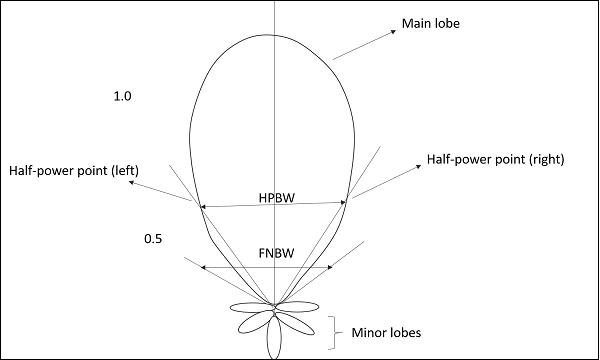# Antenna Theory – Beam Width

In this chapter, we shall discuss about another important factor in the radiation pattern of an antenna, known as beam width. In the radiation pattern of an antenna, the main lobe is the main beam of the antenna where maximum and constant energy radiated by the antenna flows.

Beam width is the aperture angle from where most of the power is radiated. The two main considerations of this beam width are Half Power Beam Width (HPBW) and First Null Beam Width (FNBW).

## Half-Power Beam Width

According to the standard definition, âThe angular separation, in which the magnitude of the radiation pattern decreases by 50% (or -3dB) from the peak of the main beam, is the Half Power Beam Width.â

In other words, Beam width is the area where most of the power is radiated, which is the peak power. Half power beam width is the angle in which relative power is more than 50% of the peak power, in the effective radiated field of the antenna.

### Indication of HPBW

When a line is drawn between radiation patterns origin and the half power points on the major lobe, on both the sides, the angle between those two vectors is termed as HPBW, half power beam width. This can be well understood with the help of the following diagram.The figure shows half-power points on the major lobe and HPBW.

### Mathematical Expression

The mathematical expression for half power beam width is –

$$Half\: power\: Beam \:with=70\lambda_{/D}$$

Where

• $\lambda$ is wavelength (Î» = 0.3/frequency).

• D is Diameter.

### Units

The unit of HPBW is radians or degrees.

## First Null Beam Width

According to the standard definition, âThe angular span between the first pattern nulls adjacent to the main lobe, is called as the First Null Beam Width.â

Simply, FNBW is the angular separation, quoted away from the main beam, which is drawn between the null points of radiation pattern, on its major lobe.

### Indication of FNBW

Draw tangents on both sides starting from the origin of the radiation pattern, tangential to the main beam. The angle between those two tangents is known as First Null Beam Width (FNBW).

This can be better understood with the help of the following diagram.The above image shows the half power beam width and first null beam width, marked in a radiation pattern along with minor and major lobes.

### Mathematical Expression

The mathematical expression of First Null Beam Width is

$$FNBW = 2 HPBW$$ $$FNBW\:2\left ( 70\lambda/D \right )\:=140\lambda/D$$

Where

• $\lambda$ is wavelength (Î» = 0.3/frequency).
• D is Diameter.

### Units

The unit of FNBW is radians or degrees.

## Effective Length & Effective Area

Among the antenna parameters, the effective length and effective area are also important. These parameters help us to know about the antennas performance.

### Effective length

Antenna Effective length is used to determine the polarization efficiency of the antenna.

Definition– âThe Effective length is the ratio of the magnitude of voltage at the open terminals of the receiving antenna to the magnitude of the field strength of the incident wave front, in the same direction of antenna polarization.â

When an incident wave arrives at the antennas input terminals, this wave has some field strength, whose magnitude depends upon the antennas polarization. This polarization should match with the magnitude of the voltage at receiver terminals.

### Mathematical Expression

The mathematical expression for effective length is –

$$l_{e} = \frac{V_{oc}}{E_{i}}$$

Where

• $l_{e}$ is the effective length.

• $V_{oc}$ is open-circuit voltage.

• $E_{i}$ is the field strength of the incident wave.

### Effective area

Definition – âEffective area is the area of the receiving antenna, which absorbs most of the power from the incoming wave front, to the total area of the antenna, which is exposed to the wave front.â

The whole area of an antenna while receiving, confronts the incoming electromagnetic waves, whereas only some portion of the antenna, receives the signal, known as the effective area.

Only some portion of the received wave front is utilized because some portion of the wave gets scattered while some gets dissipated as heat. Hence, without considering the losses, the area, which utilizes the maximum power obtained to the actual area, can be termed as effective area.

Effective area is represented by $A_{eff}$.